Pyramid
 To the Main Page    "Mathematische Basteleien"

What is a Pyramid?
 ...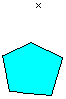... Give a plane polygon and a point, which isn't inside the plane of the point.  If you connect this point with the corners of the polygon, you get a (general) pyramid. ......

 ...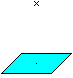... If the polygon is a square and the point lies over the centre of the square, the straight square pyramid, shortly called pyramid, develops. The following site relates to this regular pyramid. ......

Pieces of the Pyramid  top
Lengths
 ...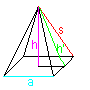... The pyramid is determined by the side a of the base and the height h.  The triangular side s and the triangular height h' are further lines.

Angles
 ...... The angle between a triangle and the base (angle of inclination) is typical for the form of the pyramid.

Surface
 ...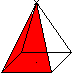...... The four lateral faces form M (M=2ah').  The lateral surface O consists of the base and the lateral faces.  (O=a²+2ah').  (German: Mantel M=coat, Oberfläche O=lateral faces)

Volume
 ...... If you put a rectangular solid with the volume a²h around the pyramid and move the vertex to the corners of the solid, you get a crooked pyramid with the same volume. There are two additional pyramids of the same volume. They all fill the solid. The volume of one pyramid is V=(1/3)*a²h. ...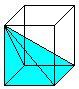...

Pyramid and Cube   top
If you succeed in making the 3D-view, you can three-dimensionally look at the following three cube pairs.
 ...... If you draw the four space diagonals of a cube, the cube is divided in six equal pyramids. The height of a pyramid is a/2. There is V=a³/6=a²*(2*h)/6=(1/3)*a²*h. This again is the well known formula for the volume of a pyramid.
The angle of inclination is 45°.

 ...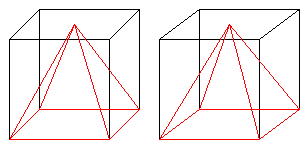... If you connect the centre of one  square and the corners of the opposite square, then a pyramid with the feature h=a develops.  The angle of inclination is arc tan(2)=63.4°.

Equilateral Pyramid    top
 ...... If all edges of a pyramid are the same (a=s) and if you reflect it at the base square, a double pyramid develops, which is only formed by equilateral triangles. This solid of eight triangles is called an octahedron. The angle of inclination of the pyramid with the equilateral triangles is  arc cos[sqrt(3)/3]= 54,7°.
The octahedron (the same as the tetrahedron, the cube, the dodecanhedron, and the isocahedron) belongs to the regular polyhedra or Platonic solids.
You can also produce an octahedron by connecting the centres of the squares of a cube by lines. Cube and octahedron are dual.

Pyramidal Numbers   top
 ...... You can build a pyramid with layers of spheres. The number of the spheres in one layer is a square number: 1,4,9,16,... , generally n². If you add the spheres layer by layer, you get the pyramidal numbers 1,5,14,30,... , generally 1+4+9+16+...+n²=n(n+1)(2n+1)/6.
In former times people kept cannon balls in such a way. They could easily count them by counting the number of the layers.

 ...... If you glue 14 marbles to two groups with six and to one pair, you get a puzzle: You must form a pyramid with three pieces.

 ...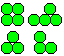... This puzzle is more complicated.  ...........................
It looks nice, if you form a pyramid with single marbles. Then the marbles in the lowest layer must lie in half rounds or in a framework.

The Great Pyramid of Gise     top
If you speak about pyramids, you usually mean the Great Pyramid, Pharao Cheop's tomb, from the 4th dynasty (2500 BC) situated 15km south of the centre of Cairo in sight of the Nile in Epypt.
Cheop's pyramid is a building with a string of superlatives: It is one of the Seven Wonders of the World, which is mainly preserved. It was the largest building up to the modern era. It is one of the most famous buildings of the world.
 ...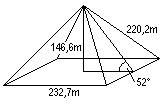... The measurements of Cheop's pyramid differ in the literature. I use the data of a new travel guide (5) hoping the last researches are considered.  Today the pyramid is 137.0m high and 230.5m long. Originally it was a little larger (on the left). It covers an area of about 5 ha. About 2,5 millions blocks with a volume of nearly 1m³ form the pyramid. The covering of polished lime plates is not preserved.

If you give the original data a=232.7m and h=146.6m, the edges are s=220.4m, the base 5.4150 ha, the lateral faces 8.7120 ha, the lateral surface 14.13 ha, the volume 2646000 m³ and the angle of inclination 51.6°.

The volume is illustrated. If you let a stone block be a cube with the edge 1m, you can form a line 2500km long. This is nearly the distance London - Athens.

Number Mysticism of the Great Pyramid   top
1
 ...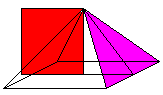... Area of the square above the height:  h² = 21780m². Area of a triangle side: (1/2)*a*h' = 21490m². Supposed: The areas are the same.  (Herodot).
The equations h²=(1/2)*a*h' and h²+a²/4=h'² (Pythagorean Theorem) lead to the ratio a:h= sqr(sqr(20)-2)=1,5723... . This is about 3.1446../2 or Pi/2.
Both equations result in the second ratio h':(a/2)=1/2*[1+sqr(5)]. This is the golden ratio phi = =1.6180... .

 ...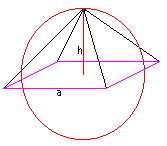... Perimeter of the square base: 4a=930.8m  Perimeter of the circle with the radius h: 2*PI*h=921.1m Supposed: The perimeters are the same.
 ...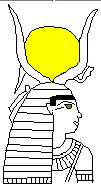...circle The ancient Egypts knew the ratio (circle perimeter :  circle diameter) as 256/81 (Rhind Papyrus 1850 BC). This leads to Pi=3.16... (book 4). The circle as a symbol for the sun was very important in the ancient Egyptian mythology and decorates the head of the Goddess Hathor (on the left) for instance. The ratio was explained on TV  by Hoimar von Ditfurth (5) this way: A circle is unrolled along a square side of Cheop's pyramid and the same circle is piled up along the height. So the ratio [perimeter : diameter]=PI appears at the pyramid.

 ...... If you lay a vertical section through the centre of a pyramid parallel to one square side, you get a (yellow) triangle. This triangle is almost the main triangle of a pentagon.  It is special that two diagonals of a pentagon divide each other in the golden ratio.
You can often see a sky with  five-cornered stars on a blue background in tombs.

 ...... The red triangle has the ratio chain  h' : h : a/2 = 5 : 3.90 : 3.11. This is nearly 5 : 4 : 3 with   5² = 4² + 3². The numbers 3,4,5 are Pythagorean numbers.  Supposed: The triangle is a Pythagorean triangle.
After the annual flood of the Nile the fields were measured by 3-4-5-strings with knots.

 ...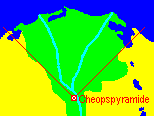... The base of the pyramid is lined up in the four points of the compass.  If you extend the diagonals of the base square, these lines include the Nile delta.
The position of  the Great Pyramid is remarkable, especially as you can look far into the Nile delta, if the smog of Cairo permits.

 ...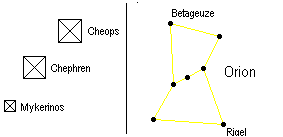... The Great Pyramid is not isolated. It nearly forms a straight line with Chefren's and Mykerinos' pyramids. The constellation Orion's three belt stars form (formed 4500 years ago) a similar line. Besides there are two more pyramids, which correspond to two corner stars of Orion. (Source: Robert Bauval, quoted in a report of the German TV station ZDF.

Summary:
It is certain, that the ancient Egyptians chose the measurements of the pyramid in order to make it safe and nice.
Who knows? Perhaps mysterious laws are hidden in the pyramids.
On the other hand: Numbers are patient... as we say in Germany.

The Power of Pyramids  top
In the American magazine "Scientific American" from June, 1974 a Dr.Matrix reported about a power, which came from pyramids. Mysterious events happened in small models of pyramids: Razor blades became sharp again, meat rotted slower, and a person sitting inside a pyramid got supernatural abilities. These statements were proved by reports from all over the world and seemed to be true.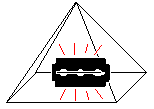Stop  ;-) !

This was a scientific joke. The yearning for esotericism, which arose at that time, should be satirized. - The famous journalist of  "Scientific American" Martin Gardner wrote this article.

I am interested, whether people sat inside small pyramids before June 1974 ;-).

Calculations     top
A pyramid is usually given by the square side a and the height h. Other pieces,  triangle side s, lateral faces M, or volume V can be calculated by them. There are the following formulas.

s²=h² + a²/2
M²=a^4 + 4a²h²
V=1/3*a²h
You can generalize: If two of the five pieces a,h,s,M, and V are given, the remaining pieces can be calculated.

There are ten cases:
1) Given: a,h. Search: V,s,M.
Solution: V=1/3a²h, s=1/2*sqr(2a²+4h²), M=a*sqr(a²+4h²).

2) Given: a,s. Search: h,V,M.
Solution: h=1/2*sqr(4s²-2a²), V=1/6*a²*sqr(4s²-2a²), M=a*sqr(4s²-a²).

3) Given:  h,s. Search: a,V,M.
Solution: a=sqr(2s²-2h²), V=2/3*h*(s²-h²), M=2*sqr(s^4-h^4).

4) Given: a,V. Search: h,s,M.
Solution: h=3V/a², s=1/2*1/a²*sqr(2a^6+36V²), M=1/a*sqr(a^6+36V²).

5) Given: h,V. Search: a,s,M.
Solution: a=1/h*sqr(3hV), s=1/2*1/h*sqr(4h^4+6hV), M=1/h*sqr(9V²+12h³V).

6) Given: s,V. Search: h,a,M
Solution: h³-s²h+3/2*V=0 und a^6-2s²a^4+18V²=0 must be solved:-(, then M=a*sqr(a²+4h²).

7) Given: a,M. Search: s,h,V.
Solution: s=1/2*1/a*sqr(M²+a^4), h=1/2*1/a*sqr(M²-a^4), V=1/6*a*sqr(M²-a^4).

8) Given: h,M. Search: s,a,V.
Solution: s=1/2*sqr[sqr(4M²-16h^4)], a=sqr[sqr(M²-4h^4)-2h²)], V=1/3*h*[sqr(M²-4h^4)-2h²].

9) Given: s,M. Search: h,a,V.
Solution: h=1/2*sqr[sqr(16s^4-4M²)], a=sqr[2s²-sqr(4s^4-M²)], dann V=1/3*a²h.

10) Given: M,V. Search: a,h,s.
Solution: a^6-M²a²+36V²=0 und 12Vh³-M²h²+9V²=0 must be solved:-(, then s=1/2*sqr(2a²+4h²).
(Thanks to 10b in 1992/93)

An Example: (Give M,V):
Which shape has a pyramid, which has the same volume and the same lateral faces as the Great Pyramid of Gise?

Solution: You derive the equation h³ - (M²/12/V)*h² + (3/4)*V = 0.
You get with V=2646000 and M=87120 the solutions h1=146.6, h2=171.4, and  h3= -79,0 (found with DERIVE).

 ...... The height h2=171.4m is the second solution.  The square side a2=215.2m belongs to the height h2.
This isn't a new contribution to the pyramid research. This only is fun.

Largest Pyramid  topYou can headfirst lay a second pyramid (green) inside a pyramid. Its vertex is in the centre of the base and its square is parallel to the base of the large pyramid. If the green pyramid is very low (1) or pointed (2), its volume is small. There is a pyramid between, which is maximum. It is the pyramid on the right (5).
You find this pyramid in the following way.
You lay a yellow triangle (3) inside the given pyramid, and introduce the square side x and the height y. The volume is V=1/3*x²y. With the help of the equation h:(h-y) = a:x you get V(x)=1/3*hx²-1/3*h/a*x³. You get x=2/3*a and further  y=1/3*h by V'(x)=0.

Pyramids on the Internet top

German

Christian Tietze/Rico Hecht
Architekturmodelle von Pyramiden
(Zur Ausstellung Pyramide  Haus für die Ewigkeit ab 6. September 2001 im Römisch-Germanischen Museum in Köln)

Frank Dörnenburg
Rätselhafte Pyramiden

Ingrid Huber  (Hubsi's Lehrer Homepage)
Grundwissen über Pyramiden

English

Andrew Bayuk  (Guardian's CyberJourney To Egypt)
The Great Pyramid

Eric W. Weisstein  (MathWorld)
Pyramid, Square Pyramid, Square Pyramidal Number

FERCO
The Pyramids of Guimar
Unter Leitung des Ethnologen Thor Heyerdahl entstand in Teneriffa ein Pyramiden-Museum. Es wird die (umstrittene) Theorie belegt, dass es einen  Zusammenhang zwischen den Pyramiden in Ägypten und in Mittelamerika gibt. Trotzdem: Ein interessantes und geschmackvoll eingerichtetes Museum.

Kevin Matthews and Artifice, Inc. (greatbuildings.com)
Sources on Great Pyramid of KhufuPyramide du Louvre

Lee Krystek
Khufu's Great Pyramid

Tim Hunkler
The Great Pyramid as Proof of God

Wikipedia
PyramidPyramid (geometry)

References    top
(1) Lancelot Hogben: Die Entdeckung der Mathematik, Stuttgart 1963
(2) Martin Gardner: Die magischen Zahlen des Dr. Matrix, Frankfurt am Main 1987
(3) Armando Curcio (Hrg.): Meilensteine der Archäologie, Herrsching 1987
(4) David Blatner: Pi, Magie einer Zahl, Reinbek bei Hamburg 1997
(5) FTI Touristik Publications: Reisebegleiter Ägypten, 2000?

(6) "Gibt es ein Geheimnis der Pyramiden?" Two TV reports from the series "Querschnitt" by Hoimar von Ditfurth, ZDF (29.03.1976 und 05.04.1976, repeated in 1991)
In these two TV reports Hoimar von Ditfurth reacted to Erich von Däniken's bestseller "Erinnerungen an die Zukunft" with the speculation: "The pyramids were built with the help of extraterrestrial beings".
The report said: "The ancient Egypts already had the abilities to build the pyramids."

I made this website after a sight-seeing tour through Epypt including the pyramids of Gise in April 2001.

Gail from Oregon Coast, thank you for supporting me in my translation.

Feedback: Email address on my main page